## Analysis of spectrum of modes during predictive modeling of DIII-D discharge 154406

The Weiland component of MMM7.1 predicts two electron and two ion modes during the evolution of temperature profiles in the DIII-D discharge 154406.

There are high frequency ion modes present at$\rho\approx 0.6$ at late stage of discharge.

## Shafranov shift scaling study for the DIII-D discharge 154406

The Shafranov shift effect is included through a geometric factor modifying the ion magnetic drift frequency, which varies strongly around each magnetic surface:$\omega_{Dj}=\displaystyle{\frac{-2k_{\theta}T_j}{Z_j e B R}} \left(\cos\theta+s\theta\sin\theta+\alpha_m\sin^2\theta\right)$

j is the ion specie index, Z is the ion charge,$k_{\theta}$ is the wave vector in the poloidal direction, s is the magnetic shear, and$\alpha_m$ is the alpha MHD which is defined as$\alpha_m=\displaystyle{\frac{2\mu_0 R q^2}{B^2}} \frac{dp}{dr}$.

The alpha MHD is proportional to the Shafranov shift and the effect of the Shafranov shift can be investigated by introducing a scaling factor for the last term in the first equation above:$\omega_{Dj}=\displaystyle{\frac{-2k_{\theta}T_j}{Z_j e B R}} \left(\cos\theta+s\theta\sin\theta+C_{\rm MMM}^{(4)}\alpha_m\sin^2\theta\right)$.

The coefficient$C_{\rm MMM}^{(4)}$ is added to the PTRANSP namelist. Five values of$C_{\rm MMM}^{(4)}$ are tested:$C_{\rm MMM}^{(4)} = 0.0$ – simulation 154406A51 (dashed curve with triangles)$C_{\rm MMM}^{(4)} = 0.5$ – simulation 154406A52 (dash-dotted curve with crosses)$C_{\rm MMM}^{(4)} = 1.0$ – simulation 154406A30 (solid curve with circles)$C_{\rm MMM}^{(4)} = 2.0$ – simulation 154406A53 (dashed curve with squares)$C_{\rm MMM}^{(4)} = 4.0$ – simulation 154406A54 (dashed curve with circles)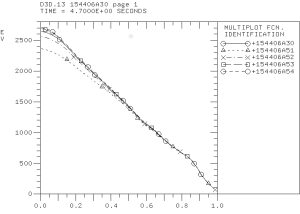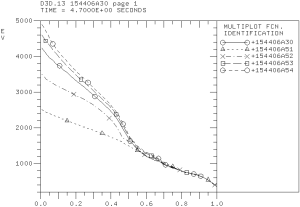The predicted electron (left) and ion (right) temperature profiles.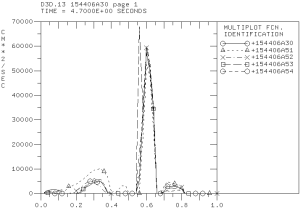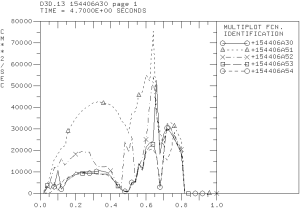Electron (left) and ion (right) thermal diffusivity profiles computed using the Weiland component of MMM7.1.

The Shafranov shift has stabilizing effect on electron and ion channels of transport. However, the effect on the electron channel is somewhat weaker. Also, the large spike in transport in the ITB region in the electron thermal diffusivities is found weakly affected by the Shafranov shift.

## Evolving the equilibrium in the simulation of DIII-D discharge 154406

Previous simulation used prescribed time-dependent equilibrium quantities that are based on the experimental equilibrium reconstruction. Using TEQ equilibrium solver in PTRANSP eliminates additional constrains on predicted profiles. The figure below shows the predicted and reconstructed q-profiles at 5.2 sec. The q-profile from experimental has a local minimum in the region of internal transport barrier. The predicted q-profile is significantly modified with the local minimum shifted towards magnetic axis.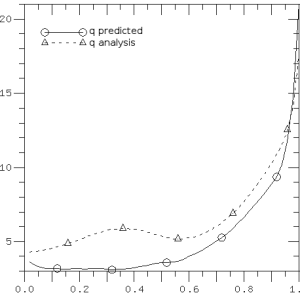## More on the ExB flow shear effect on the temperature prediction for the DIII-D discharge 154406

The PTRANSP simulation 1554406A44 with the ten times ExB flow shear scaling factor (FACEXB=10) is completed. A notable effect on the electron and ion temperatures is observed. The temperatures are higher comparing to the previous simulations with FACEXB=0 (154406A41), FACEXB=1 (154406A30), and FACEXB=2 (154406A38). The main conclusion from these simulations is that the experimental level of ExB flow shear is low enough to have any significant effect on the anomalous transport.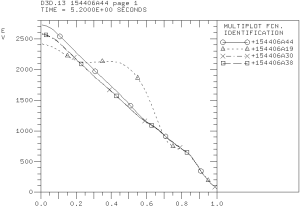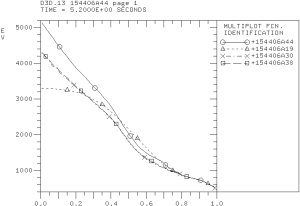## Role of ExB flow shear on the prediction of temperature profiles for the DIII-D discharge 154406

The role of the ExB flow shear on the predicted temperature profiles for the DIII-D discharge 154406 is investigated using the FACEXB scaling factor. I noticed a very small change in the predicted temperatures when this factor is set to 0 (154406A41), 1 (154406A30), and 2 (154406A38). Another simulation (154406A44) with the FACEXB factor set to 10 is also started.

## Investigation of the boundary condition effect on the electron temperature prediction for the DIII-D discharge 154406

The boundary conditions have been moved from 0.8 to 0.9 in the simulation of the electron temperature profile for the DIII-D discharge 154406 (154406A40). The ion temperature is not evolved. The predicted temperature profile agree with the experimental profile at approximately the same level as in the simulation with the boundary condition set at 0.8 (154406A30).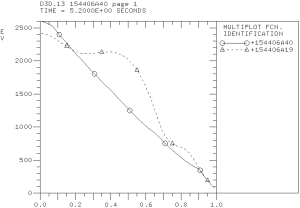The predicted electron temperature profile shown as a solid curve is compared with the experimental temperature profile shown as dashed curve.

## Contributions from the drift resistive ballooning modes to the temperature prediction for the DIII-D discharge 154406

The drift resistive inertial ballooning modes (DRIBM) are destabilized by the density gradients. These modes are strongly stabilized by plasma temperatures. They are usually unstable in the plasma edge region close to the separatrix. The DRIBM modes are expected to be stable in the plasma core region of H-mode discharges. However, PTRANSP analysis of the DIII-D discharge 154406 shows that these modes are unstable in the region from 0.3 to 0.7 of normalized minor radius: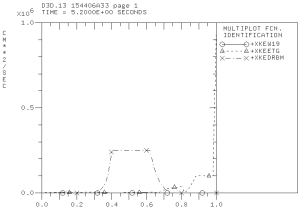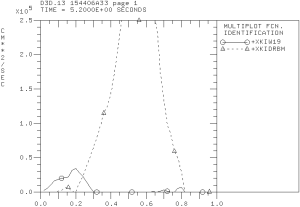Effective electron (left) and ion (right) thermal diffusivities computed using the MMM7.1 model in the modeling of DIII-D discharge 154406 by the PTRANSP code.

The DRIBM model includes contributions from other MHD modes in addition to the drift resistive ballooning modes that can be unstable in this region. However, the validation of predicted temperature profiles hints that these modes are probably should be stable and DRIBM predicts significantly larger level of transport than expected. Additional validation of DRIBM is necessary. In particular, the effects associated with the large poloidal beta or large plasma currents such as Shafranov shift stabilization in DRIBM might need to be revisited.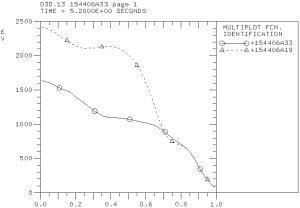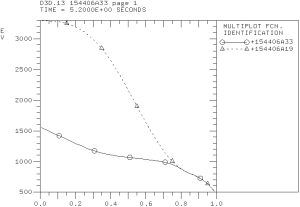Predicted electron (left) and ion (right) temperature profiles in the PTRANSP simulation of the DIII-D discharge 154406 using the Weiland, ETG, and DRIBM components of the MMM7.1 model.

## Predicting the temperature profiles for the DIII-D discharge 154406

The temperature profiles are predicted using the Weiland and ETG components of the Muti-Mode Model (MMM7.1). The predicted temperature profiles are compared with the corresponding temperatures from interpretive simulations (154406A19). Four PTRANSP simulations are completed. These simulations are: (1) Only electron temperature is evolved (154406A28); (2) Only ion temperature is evolved (154406A29); (3) Electron and ion temperatures are evolved (154406A30); and (4) Electron and ion temperature as well as toroidal momentum are evolved (154406A31).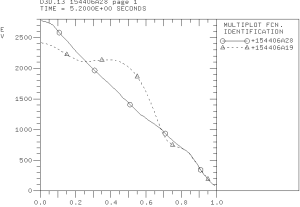The electron temperature profile in the PTRANSP simulation 154406A28.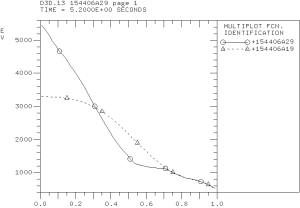The ion temperature profile predicted in the PTRANSP simulation 154406A29.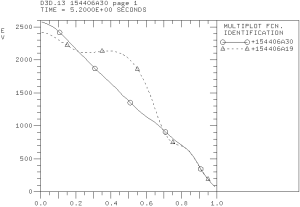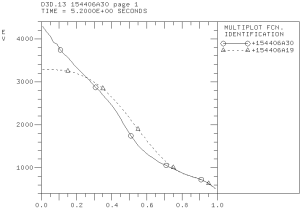The electron (left) and ion (right) temperature profiles predicted in the PTRANSP simulation 154406A30.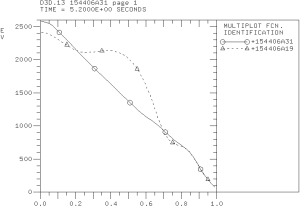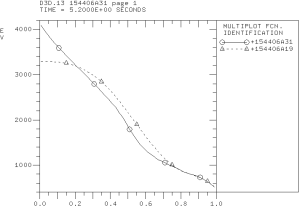The electron (left) and ion (right) temperature profiles predicted in the PTRANSP simulation 154406A31.

It is somewhat unexpected that a that better agreement between predicted and experimental profiles is found in the simulations in which the electron and ion temperatures are evolved simultaneously. Neither simulation can accurately reproduce the location of internal transport barrier in the electron temperature profile. The reason is a rather significant electron thermal transport from both the Weiland component (TEM and ITG modes) and ETG component of MMM7.1 that destroys ITB from strong off-axis ECR heating.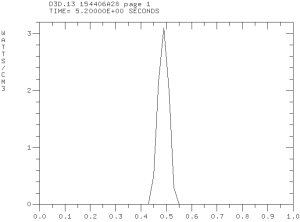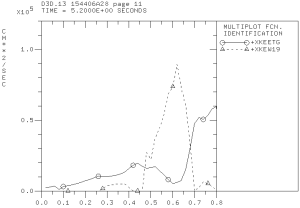The ECR heating profile (left) and electron thermal effective diffusivities (right) computed in the Weiland and ETG components of MMM7.1 in the predictive PTRANSP simulation 154406A28.

## Simulation of high poloidal beta DIII-D discharge 154406

The TRANSP code is used to simulated the high poloidal beta DIII-D discharge 154406. Previous simulations that were presented by Andrea Garofalo at the TTF meeting (April 28-May 1, 2015; Salem, MA) and the H-mode workshop (October, 2015; Garching, Germany) includes the results obtained by Gary Staebler and Brian Grierson using the GLF23 and TGLF models. The ion thermal transport is found close to the neoclassical level. The electron temperature is found to be over-predicted. My new TRANSP simulations are started from the existing TRANSP run 154406R04 on the PPPL cluster. A number of simulations using various MMM7.1 parameters are performed. Both electron and ion temperatures are found to be strongly under-predicted. I have also generated new TRANSP input files using AUTOTRANSP. Some NUBEAM and ECH parameters are found different from the corresponding parameters in the 154406R04TR.DAT file. These differences resulted in significant differences in the total fast ion heating BPHTO which is related to anomalously large outside CX losses BPCXX in the simulations that are based on the run 154406R04.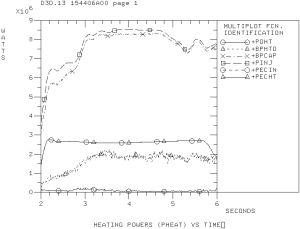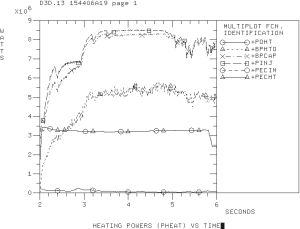Input heating powers in the simulation that is based on input from the 154406R04 input (left) and autotransp (right).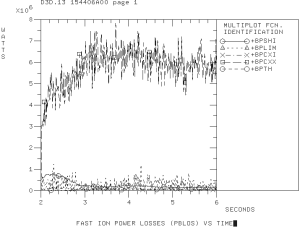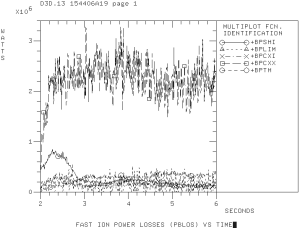Beam losses in the simulation that is based on the 154406R04 input (left) and autotransp (right).

## XGC-A07: new equilibrium and updated version of the code

The FMCFM changes has been synchronized with the version of the XGC-0 code from the svn repository. Gunyoung committed his changes related to the impurity radial model and get_mid_r bug. Theory motivated coefficient for the flow shear suppression factor is used. The simulation uses high resolution equilibrium provided by Rich Groebner. There are significant changes in the plasma density dynamics. It is not clear if these changes relate to (1) model for flow shear suppression factor; (2) new equilibrium; (3) other changes to the code. In order to isolate the problem, two new simulations are submitted: (1) XGC-A08: same equilibrium and same input file, but older version of the code; (2) XGC-A09: same equilibrium, newer version of the code, but older version of the flow shear suppression model.